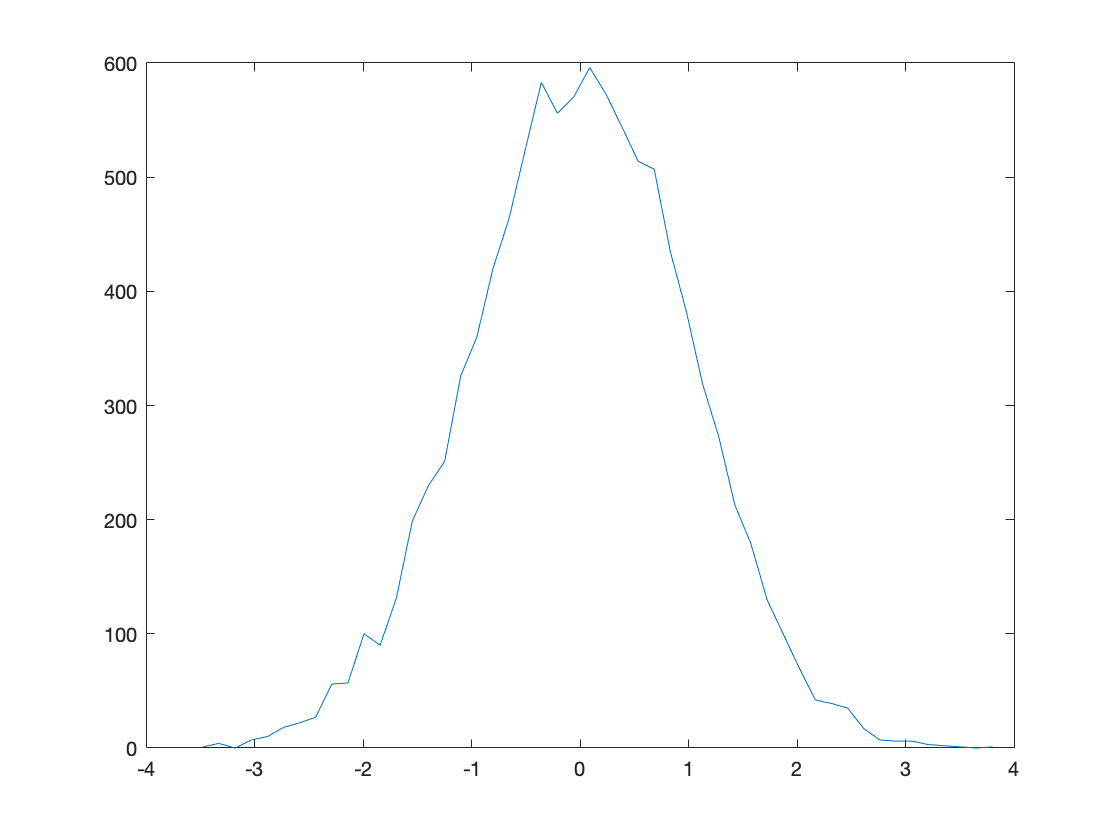# NSCS, Week 3: Matlab Concepts

## While loops

Sometimes we want to repeat a section of code until some condition is met. In the perceptual decision making model this week, we wanted the code that counted the dots to continue until the threshold was crossed. For loops can't do this because they require us to specify how many times the loop should run before hand. Instead we use while loops ... here's an example
% initialize the condition required to continue the while loop to true
flag = true;
% initialize a counter so we can see how many times the loop runs
i = 0;
% start the loop
while flag
% increment the counter
i = i + 1;
% generate a random number between 0 and 1
r = rand;
% if the random number is bigger than 0.9 ...
if r > 0.9
% ... set the flag to false and end the while loop
flag = false;
end
end
i
i = 14
In this example, we run a loop until a random number is bigger than 0.9. Every time you run it, this code will run the loop a different number of times, because the stopping condition is random.
Another way to say the same thing, without explicitly refering to the flag variable or the r variable would be
i = 0;
while rand < 0.9
i = i + 1;
end
i
i = 5
Everytime through the loop, the while line generates a new random number and checks whether it is less than 0.9. If it is, then the loop continues. If it isn't, then the loop stops.

## Plotting histograms with hist

The hist function plots a histogram. For example, if we want to see what random numbers from the randn function look like we could do this ...
for i = 1:10000
r(i) = randn;
end
hist(r)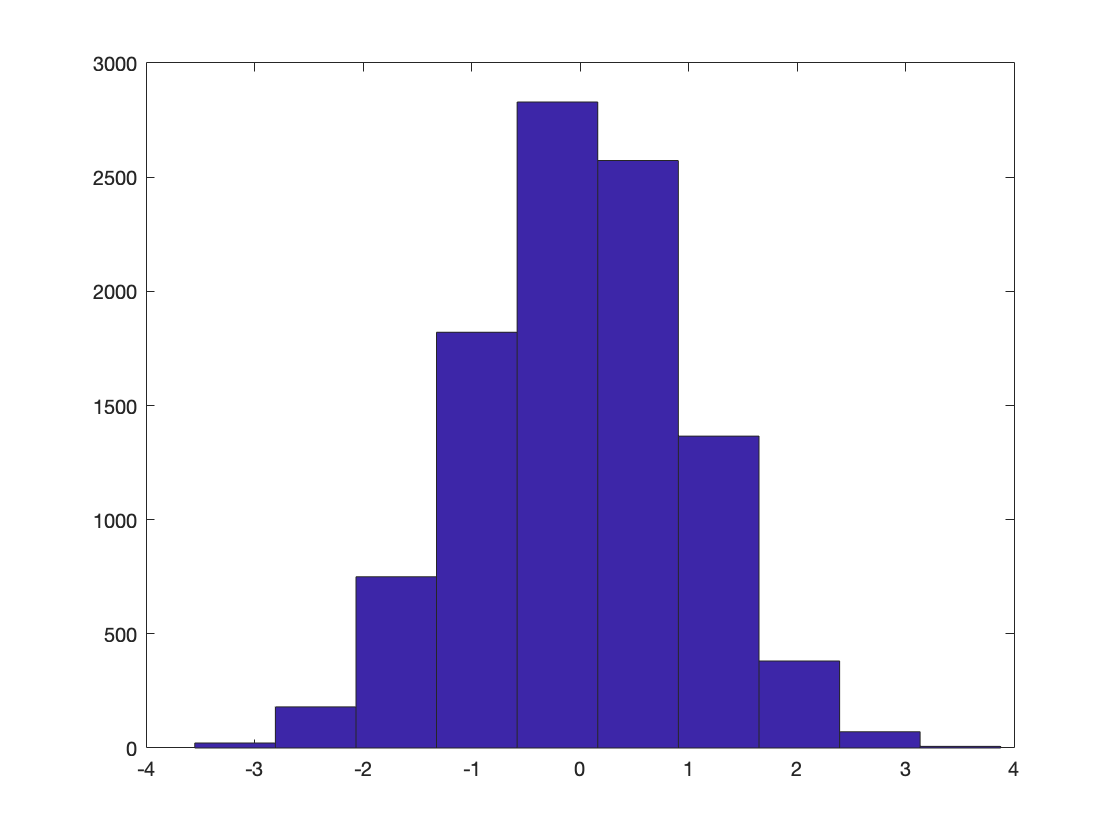randn generates a random number from a Gaussian distribution with mean 0 and standard deviation 1. The histogram looks consistent with this.
With default settings, hist only uses 10 bins. If we'd like a better defined histogram we can specify the number of bins with a second argument ...
hist(r, 20)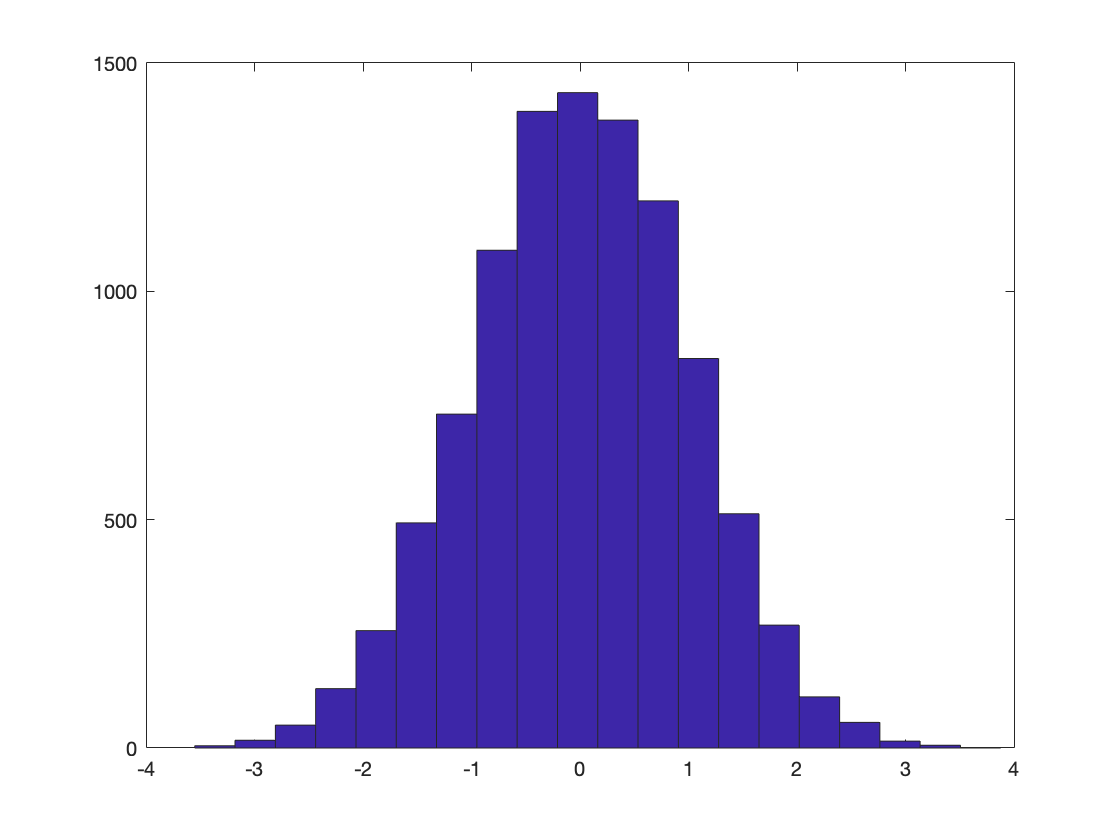or
hist(r,50)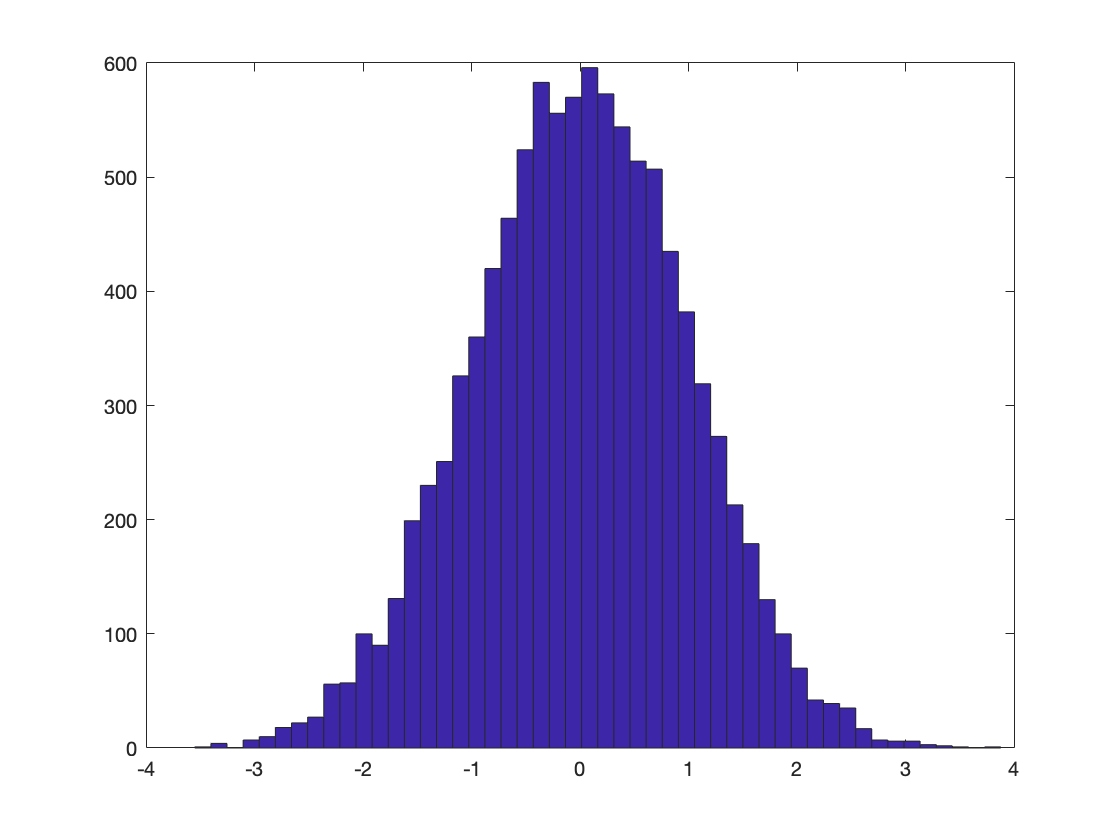If we'd like to explicitly define the bins we can make the second argument a vector of bin edges
hist(r, [-4:1:4])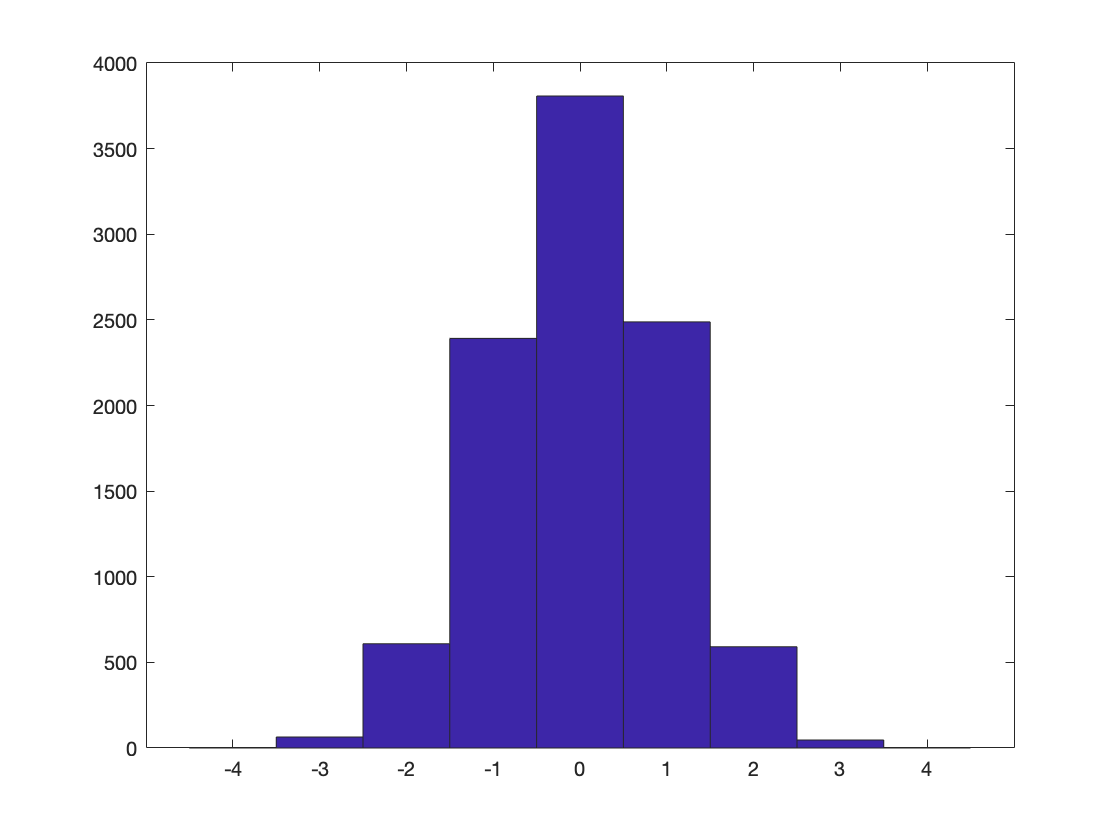This gives us bin edges at whole numbers.
If we want to get the bar heights and bin centers out as variables, we can write
[barHeights, binCenters] = hist(r, 50)
barHeights = 1×50
1 4 0 7 10 18 22 27 56 57 100 90 131 199 230 251 326 360 420 464 524 583 556 570 596 573 544 514 507 435 382 319 273 213 179 130 100 70 42 39 35 17 7 6 6 3 2 1 0 1
binCenters = 1×50
-3.4764 -3.3278 -3.1792 -3.0307 -2.8821 -2.7335 -2.5849 -2.4364 -2.2878 -2.1392 -1.9906 -1.8421 -1.6935 -1.5449 -1.3963 -1.2478 -1.0992 -0.9506 -0.8020 -0.6535 -0.5049 -0.3563 -0.2077 -0.0591 0.0894 0.2380 0.3866 0.5352 0.6837 0.8323 0.9809 1.1295 1.2780 1.4266 1.5752 1.7238 1.8723 2.0209 2.1695 2.3181 2.4666 2.6152 2.7638 2.9124 3.0609 3.2095 3.3581 3.5067 3.6553 3.8038
Note this doesn't plot anything, but does give us numbers that we can use to plot the histogram either as a bar plot (using the bar function) or a line plot (using the plot function)
bar(binCenters, barHeights)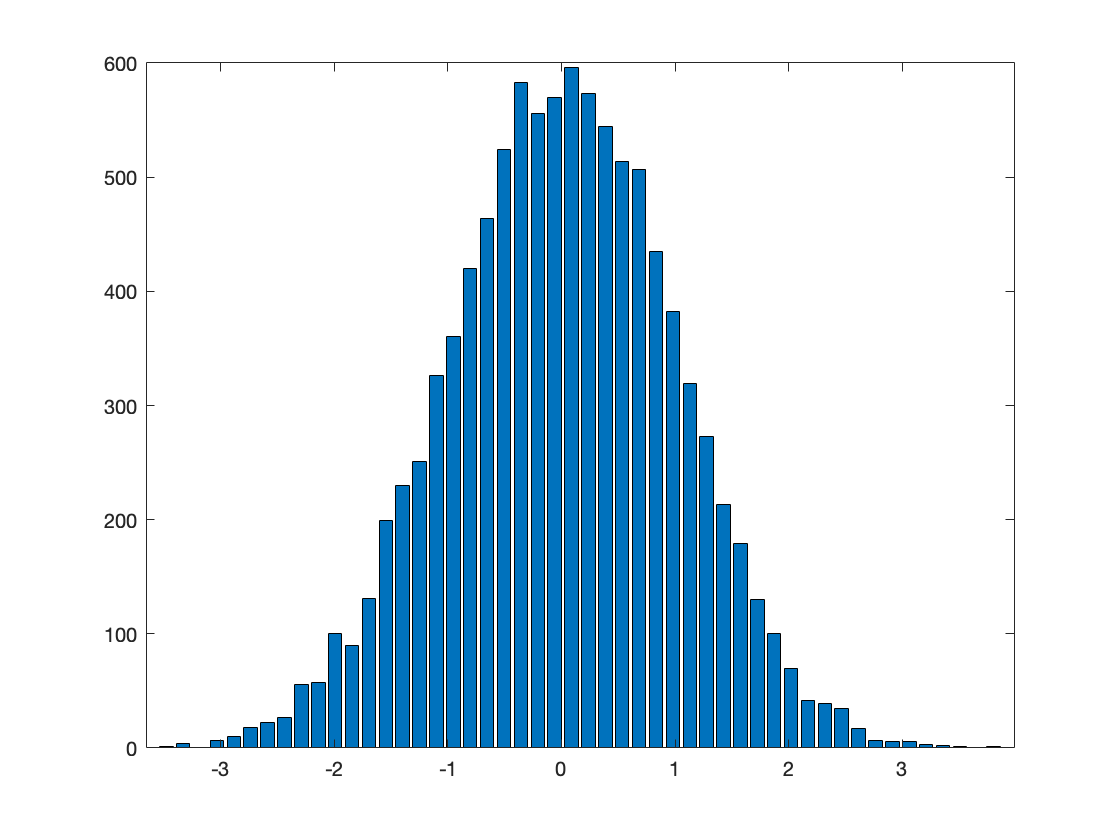plot(binCenters, barHeights)